Get instant live expert help with Excel or Google Sheets“My Excelchat expert helped me in less than 20 minutes, saving me what would have been 5 hours of work!”

#### Post your problem and you’ll get expert help in seconds.

Your message must be at least 40 characters
Our professional experts are available now. Your privacy is guaranteed.

# How to Use Excel VBA String Functions – Excelchat

When working with strings in VBA, we need to know how to manipulate those string functions. A String may be a sequence of letters, numbers or other characters stored as a text. In this tutorial, we will learn how to use VBA string functions like LEFT, RIGHT, MID, LEN, REPLACE, INSTR, LTRIM, SPLIT, and STRCOMP.

## The syntax for the VBA string function is:

`String (Number, Character)`

Where

• Number – the number of characters in the returned string
• Character – is the character code or a string representing the character to be repeated in the returned string.

## Excel VBA String functions

Many string functions can be used in VBA and in the worksheet. However, we will concentrate on VBA string functions, which can or cannot be used in a worksheet.

## Preparing to run VBA Excel String manipulations

We will create a command button to help understand how this VBA string functions work.

• We will go to the Developer tab and select Command button under the ActiveX Controls group.

##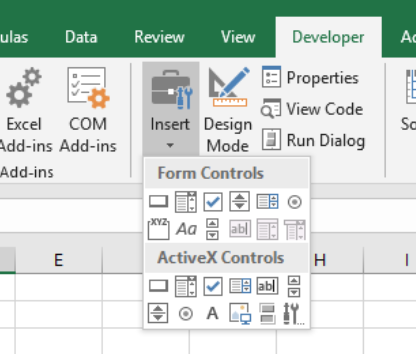Figure 1 – Using VBA string functions

• Next, we will drag to draw the command button on our excel worksheet.

##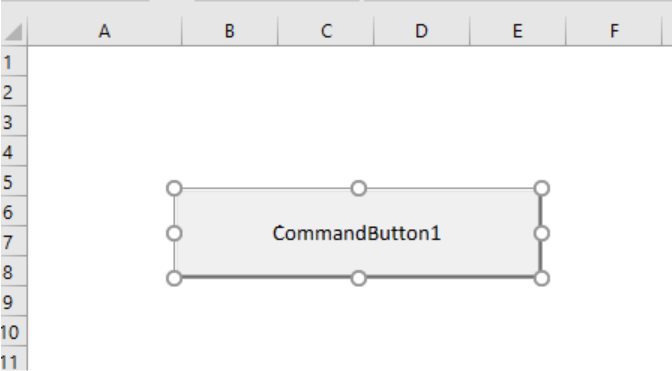Figure 2 – Assigning Macro for VBA Strings

• To Assign Macro, we will make sure the Design mode is selected. Next, we will right click on the button and click on View Code. In the Microsoft Visual Basic window, we will be adding the codes from the following VBA string function examples.

## How to extract part of a string

We use the Left, Right and Mid functions in extracting parts of a string. In this section, we will run the Left, Right and Mid functions on the word “John Magnus Smith”.

### Left

We use the Left function to extract a substring beginning from the left side of the string.

`Dim text As String`

`text = "John Magnus Smith"`

`MsgBox Left(text, 4)`

##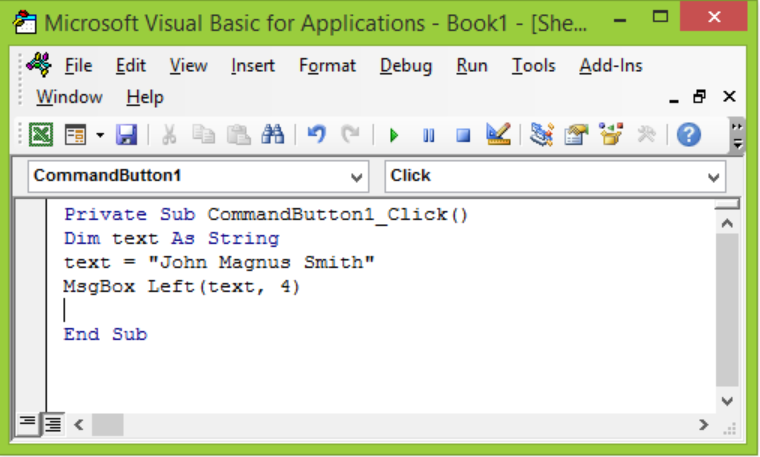Figure 3 – Using Substrings in VBA

##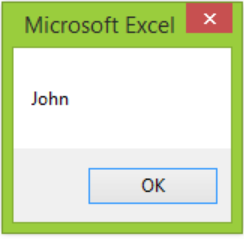Figure 4 – Results

### Right

We use the Right VBA string function to extract a sub-string beginning from the right side of the string.

`Dim text As String`

`text = "John Magnus Smith"`

`MsgBox Right(text, 4)`

##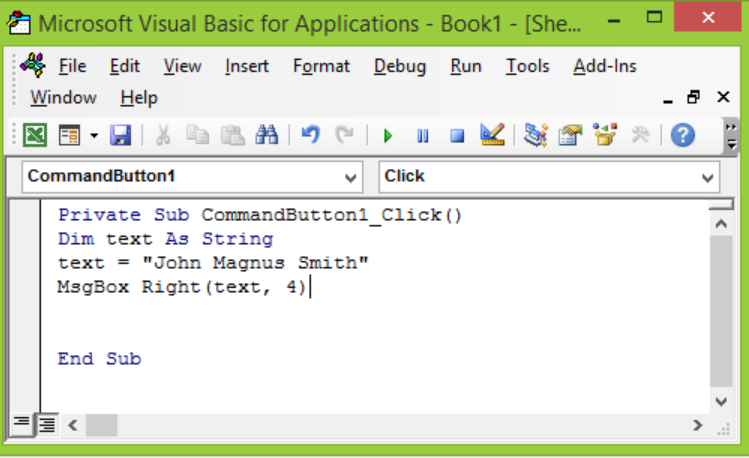Figure 5 – Using VBA strings

##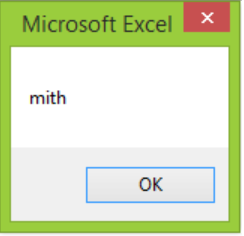Figure 6 – Using VBA String Manipulations

### Mid Function

We can use the Mid string function to extract a substring beginning from the middle as specified by our VBA command.

`Dim text As String`

`text = "John Magnus Smith"`

`MsgBox Mid(“John Magnus Smith”, 9, 4)`

##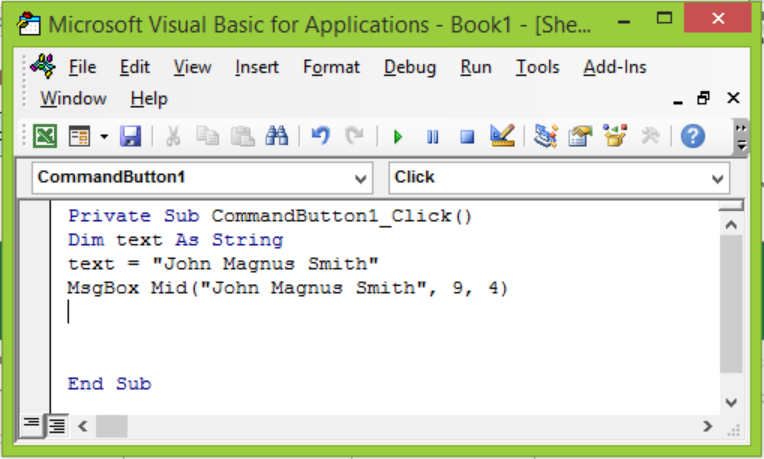Figure 7 – VBA String functions

##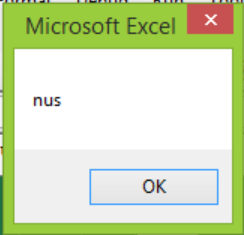Figure 8 – Running VBA Text

## Building String using the Join function

There are many ways to use the Join function. We will explain two popular ways.

1. We can use the & operator to join or concatenate strings.

`Dim text1 As String, text2 As String, text3 As String`

`text1 = "Jane"`

`text2 = "Tim"`

`text3 = ”Paul”`

`MsgBox text1 & " " & text2 & “ “ & text3`

##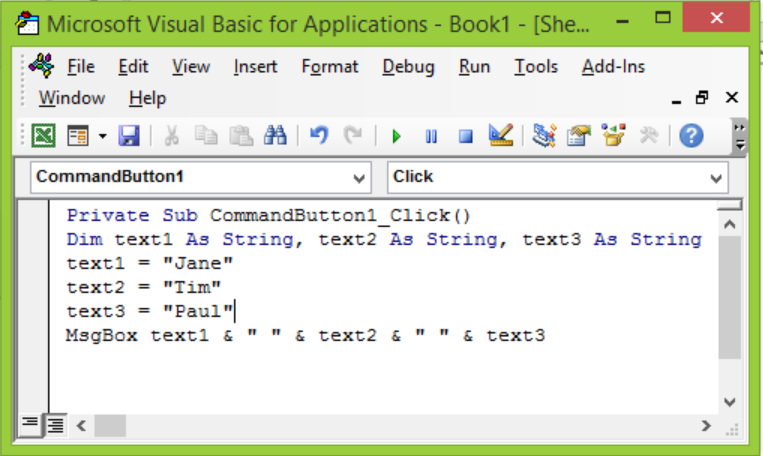Figure 9 – VBA String

##Figure 10 – Substrings in VBA

1. We can also join to create one string by specifying a delimiter (“,”) as the second argument to the function.

`Dim myDays(2) As String`

`    myDays(0) = "Mon"`

`    myDays(1) = "Tue"`

`    myDays(2) = "Wed"`

`MsgBox Join(myDays, ",")`

##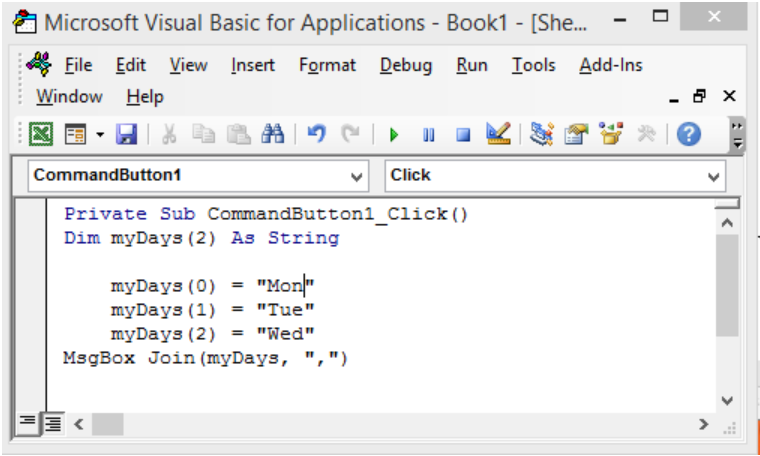Figure 11 – Joining VBA Strings

##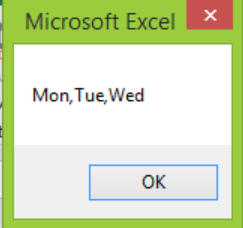Figure 12 – VBA texts

## Search within a string

We can use the InStr and InStrRev a string into multiple strings each stored as an element in an array.

### InStr

We can use the InStr to find the position of the specified text from the beginning.

`Sub FindSubString()`

`    Dim name As String`

`    name = "John Smith"`

`MsgBox InStr("John Smith", "Sm")`

`End Sub`

##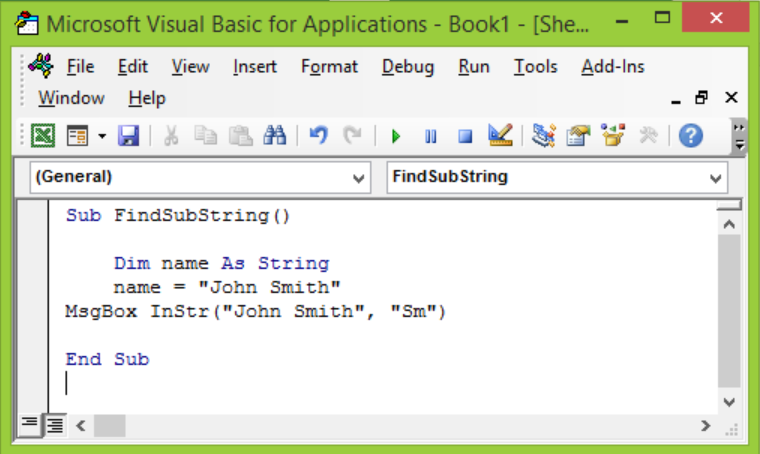Figure 13 – VBA find text in string

##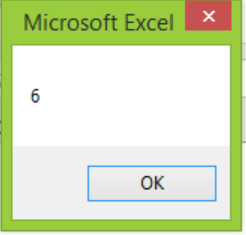Figure 14 – Search Results

### InStr Rev

Alternatively, we use the Instr Rev to search from the specified position. However, the InstrRev function can return the same value as the Instr because it searches from the end and then returns to begin from the start position.

`Sub UsingInstrRev()`

`    Dim name As String`

`    name = "John Magnus Smith"`

`MsgBox InStrRev("John Magnus Smith", "t")`

`End Sub`

##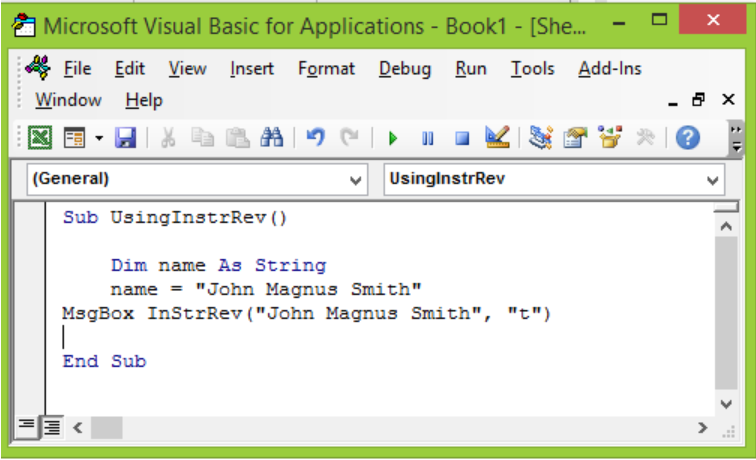Figure 15 – VBA in Strings

##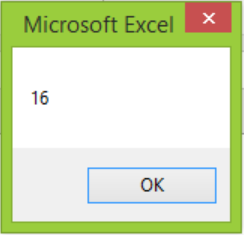Figure 16 – Results

## Replace part of a string

We can use the Replace VBA string function to replace one substring with another substring.

`Sub ReplaceExamples()`

`text = "John Magnus Smith"`

`MsgBox Replace(“John? Magnus? Smith”, “?”,  “;”)`

`End Sub`

##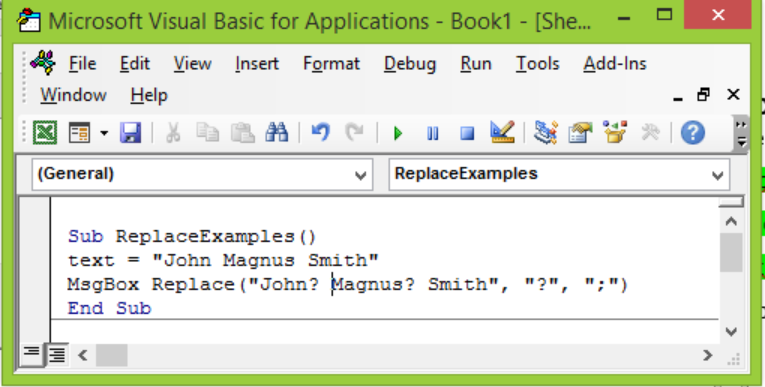Figure 17 – VBA texts

##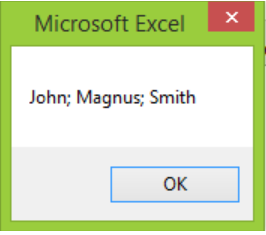Figure 18 – Substring in VBA functions

## Making Substitutions

### Replace

We use the Replace function to make substitutions within a string of characters.

`Sub ReplaceExamples()`

`text = "John Magnus Smith"`

`MsgBox Replace("John? Magnus? Smith", "?", ";")`

`End Sub`

##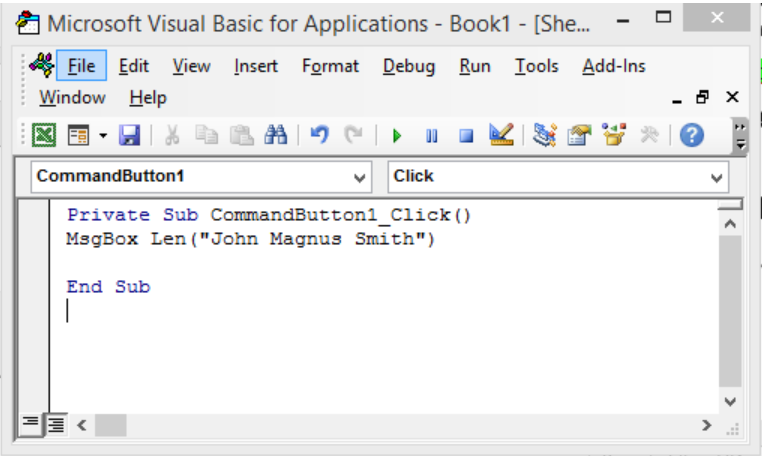Figure 19 – Excel As String in VBA

##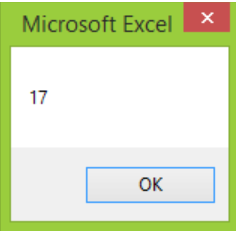Figure 20 – Substrings in VBA

### ReplaceCount

We can equally make substitutions and specify the number of substitutions we want using the Replace Count.

`Sub ReplaceCount()`

`text = "John Magnus Smith Sophie Emma"`

`MsgBox Replace("John? Magnus? Smith", "?", ";" , Count: =3)`

`End Sub`

##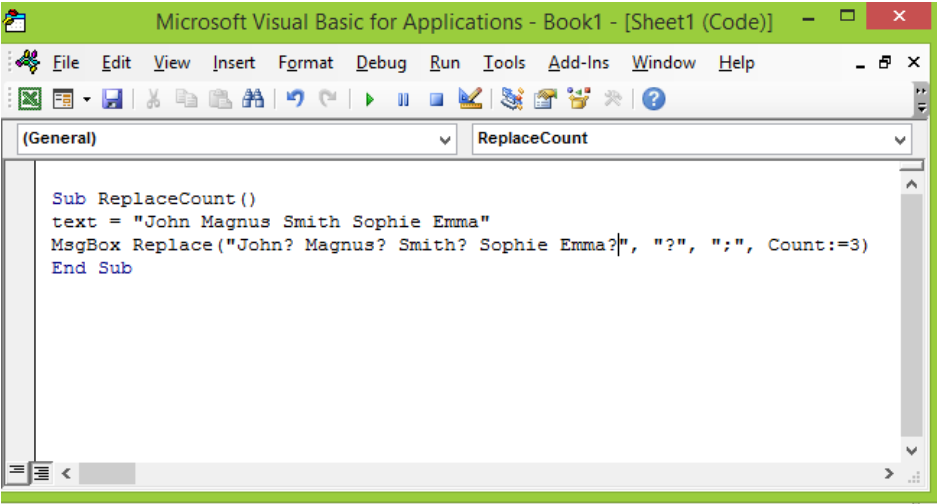Figure 21 – VBA string functions

##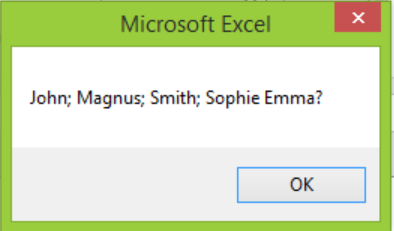Figure 22 – Replaced Text in VBA string

## Making Comparisons

We can use VBA string functions to make a wide range of comparisons. We will make comparisons using StrComp and operators.

### Comparing Two Strings using StrComp

Syntax: StrComp()  String1, String2, Compare[Optional]

When we use the StrComp function, our results may yield the following:

Return Value               Description

0                                 Strings match

1                                 String1 greater than String2

-1                                String1 less than string2

`Null if either string is null`

`Sub UsingStrComp()`

`text = "John Magnus Smith Sophie Emma"`

`MsgBox StrComp("John”, “James”, vbTextCompare)`

`End Sub`

##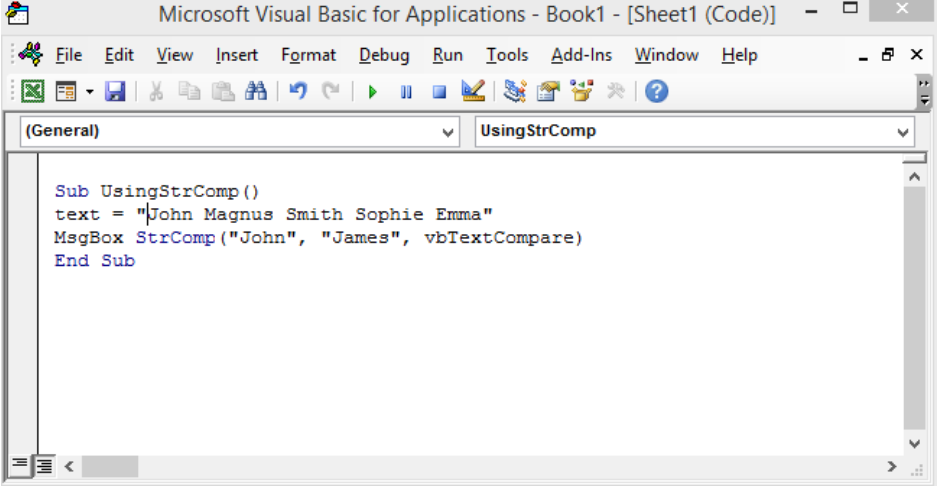Figure 23 – VBA Strings

##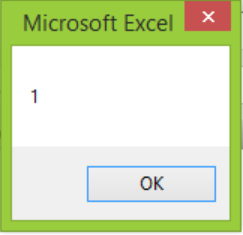Figure 24 – Results

### Compare String using Operators

We can either use the equals sign (=) or <> to compare strings.

1. With the equals sign, we may have a true or false result.

`Sub CompareUsingEquals()`

`text = "John =James"`

`MsgBox  ("John” = “James”)`

`End Sub`

##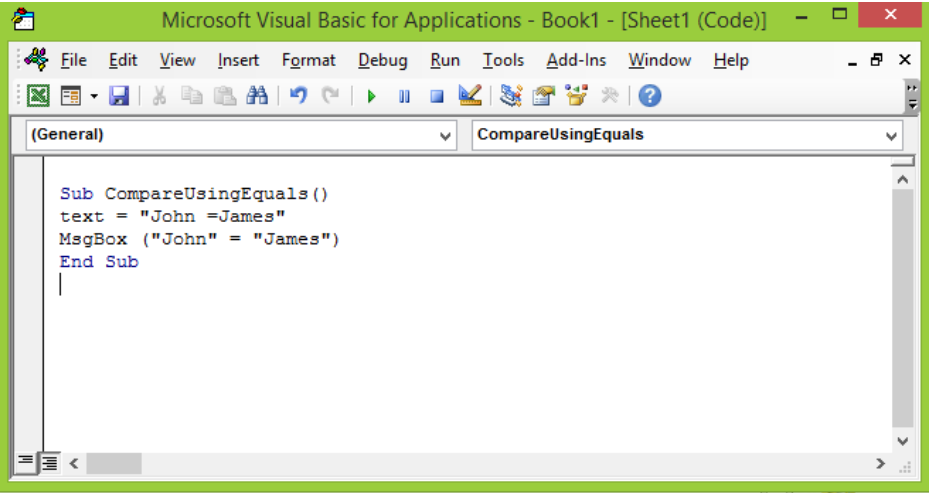Figure 25 – Substrings in VBA

##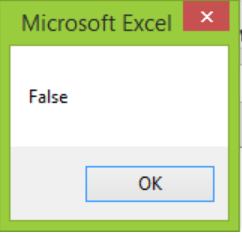Figure 26 – VBA Text Result

2. Alternatively, we can use the <> (does not equal). Our results will be True or False as expressed earlier.

`Sub CompareWithNotEqual()`

`text = "John  < > James"`

`MsgBox  ("John” < > “James”)`

`End Sub`

##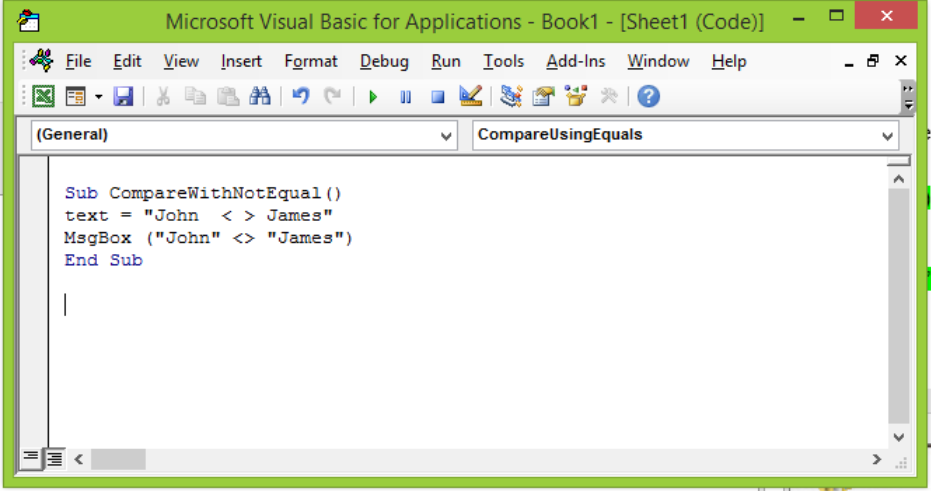Figure 27 – VBA text

##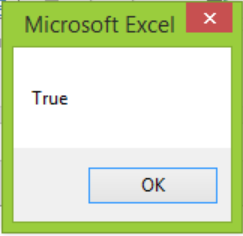Figure 28 – Substrings in VBA

## Removing Blanks with VBA String functions

We use the LTrim, RTrim and Trim VBA functions to remove spaces from the start or end of our string.

### LTrim

We use the LTrim removes spaces beginning from the left of our string

`Sub LTrimStr()`

`Dim text As String`

`text = "John Magnus Smith  "`

`MsgBox LTrim(“John Magnus Smith  “)`

`End Sub`

##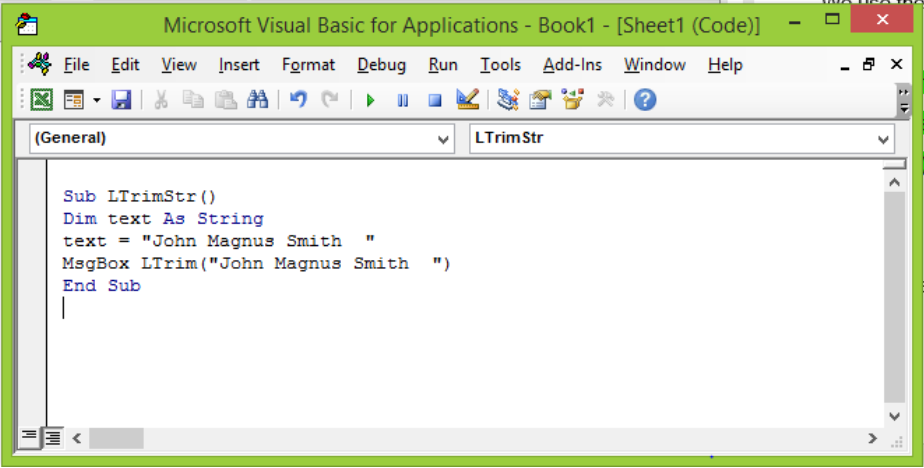Figure 30 – Removing blanks in VBA strings

##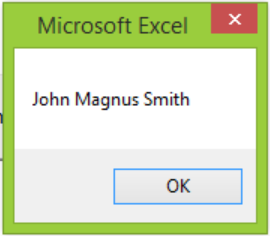Figure 31 – Results.

### RTrim

RTrim removes spaces beginning from the right of the string

`Sub RTrimStr()`

`Dim text As String`

`text = "   John Magnus Smith  "`

`MsgBox LTrim(“   John Magnus Smith  “)`

`End Sub`

##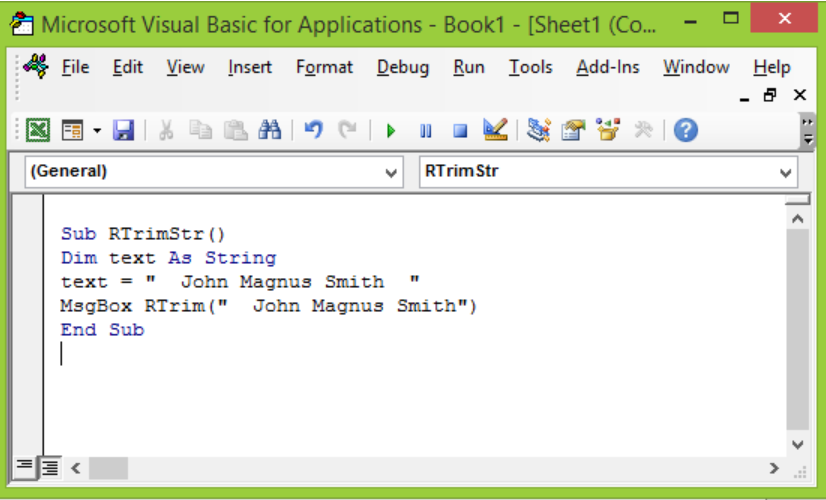Excel 32 – Using VBA text as String

##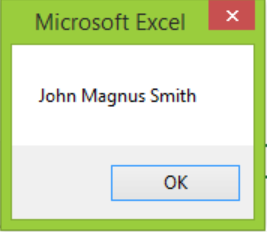Excel 33 – VBA Strings

### Trim

Trim removes spaces from the left and right of a string.

`Sub TrimStr()`

`Dim text As String`

`text = "  John Smith  "`

`MsgBox Trim("  John Smith ")`

`End Sub`

##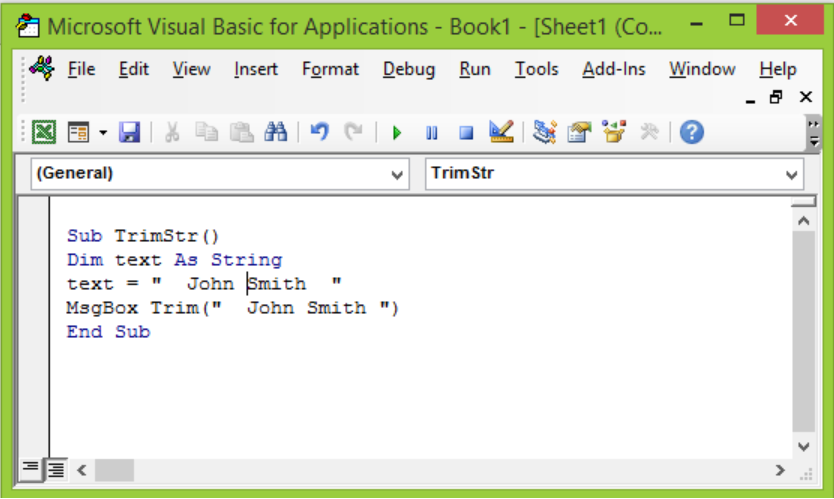Figure 34 – VBA Strings

##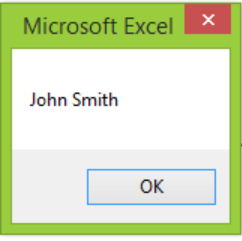Figure 35 – Results

## Note

We do not use VBA String functions to change the original string. They will simply return with a new string alongside the changes we made in the function. If we want to change the original string, we simply transform the result to the original string.

## Instant Connection to an Excel Expert

Most of the time, the problem you will need to solve will be more complex than a simple application of a formula or function. If you want to save hours of research and frustration, try our live Excelchat service! Our Excel Experts are available 24/7 to answer any Excel question you may have. We guarantee a connection within 30 seconds and a customized solution within 20 minutes.